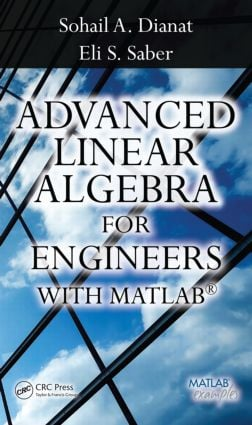Advanced Linear Algebra for Engineers with MATLAB

1st Edition

CRC Press

346 pages | 3 Color Illus. | 73 B/W Illus.

Purchasing Options:\$ = USD
Hardback: 9781420095234
pub: 2009-02-23
SAVE ~\$28.00
\$140.00
\$112.00
x
eBook (VitalSource) : 9781315218489
pub: 2017-12-19
from \$70.00

FREE Standard Shipping!

Description

Arming readers with both theoretical and practical knowledge, Advanced Linear Algebra for Engineers with MATLAB® provides real-life problems that readers can use to model and solve engineering and scientific problems in fields ranging from signal processing and communications to electromagnetics and social and health sciences.

Facilitating a unique understanding of rapidly evolving linear algebra and matrix methods, this book:

• Outlines the basic concepts and definitions behind matrices, matrix algebra, elementary matrix operations, and matrix partitions, describing their potential use in signal and image processing applications
• Introduces concepts of determinants, inverses, and their use in solving linear equations that result from electrical and mechanical-type systems
• Presents special matrices, linear vector spaces, and fundamental principles of orthogonality, using an appropriate blend of abstract and concrete examples and then discussing associated applications to enhance readers’ visualization of presented concepts
• Discusses linear operators, eigenvalues, and eigenvectors, and explores their use in matrix diagonalization and singular value decomposition
• Extends presented concepts to define matrix polynomials and compute functions using several well-known methods, such as Sylvester’s expansion and Cayley-Hamilton
• Introduces state space analysis and modeling techniques for discrete and continuous linear systems, and explores applications in control and electromechanical systems, to provide a complete solution for the state space equation
• Shows readers how to solve engineering problems using least square, weighted least square, and total least square techniques
• Offers a rich selection of exercises and MATLAB® assignments that build a platform to enhance readers’ understanding of the material

Striking the appropriate balance between theory and real-life applications, this book provides both advanced students and professionals in the field with a valuable reference that they will continually consult.

Matrices, Matrix Algebra, and Elementary Matrix Operations

Basic Concepts and Notation

Matrix Algebra

Elementary Row Operations

Solution of System of Linear Equations

Matrix Partitions

Block Multiplication

Inner, Outer, and Kronecker Products

Determinants, Matrix Inversion and Solutions to Systems of Linear Equations

Determinant of a Matrix

Matrix Inversion

Solution of Simultaneous Linear Equations

Applications: Circuit Analysis

Homogeneous Coordinates System

Rank, Null Space and Invertibility of Matrices

Special Matrices with Applications

Linear Vector Spaces

Linear Vector Space

Span of a Set of Vectors

Normed Vector Spaces

Inner Product Spaces

Orthogonality

Matrix Factorization

Eigenvalues and Eigenvectors

Matrices as Linear Transformations

Eigenvalues and Eigenvectors

Matrix Diagonalization

Special Matrices

Singular Value Decomposition (SVD)

Numerical Computation of Eigenvalues and Eigenvectors

Properties of Eigenvalues and Eigenvectors of Different

Classes of Matrices

Applications

Matrix Polynomials and Functions of Square Matrices

Matrix Polynomials

Cayley–Hamilton Theorem

Functions of Matrices

The State Space Modeling of Linear Continuous-time Systems

State Space Representation of Discrete-time Systems

Controllability of LTI Systems

Observability of LTI Systems

Introduction to Optimization

Stationary Points of Functions of Several Variables

Least-Square (LS) Technique

Total Least-Squares (TLS)

Eigen Filters

Stationary Points with Equality Constraints

Appendix A: The Laplace Transform

Appendix B: The z-Transform

Bibliography

Index